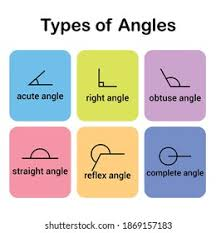On the basis of measurement, there is a various classification of the angles. We can define an angle as a geometrical shape that is formed due to the joining of rays in the ending points. The measurement of angles is usually carried out in terms of degrees. The types of angles are classified on the basis of magnitude and on basis of rotation. Generally, there are six types of angles, acute angle, obtuse angle, right angle, straight angle, reflex angle, and full rotation angle. In this article, we will try to cover and understand these angles in a detailed manner and see the comparison between them.

## Vertically Opposite Angles

Any angle which is formed when two vertical or horizontal lines meet each other at one single point is known as vertically opposite angles. When any two lines intersect with each other, it results in the formation of four angles. These pairs of angles can be considered as vertically opposite angles. These angles are always equal, can be both supplementary and complementary, and are always non-adjacent. This pair of angles always results in the formation of the alphabet ‘x’. This will also help you to recognize a pair of vertically opposite angles.

## Definition of Various Types of Angles

As mentioned above in the article, there are various types of angles categorized on the basis of magnitude and rotation. The following points mentioned below analyses the definition of various types of angles:

1. Any kind of angle that is smaller or measures less than 90 degrees is known as an acute angle. In simple words angles such as 60 degrees, 45 degrees, 30 degrees, 86 degrees, etc are examples of an acute angle. You might have observed various objects such as a piece of watermelon, a beak of a bird, the mouth of a crocodile, etc, all these are examples of an acute angle in our everyday life.
2. In a generic way, an obtuse angle is also known as a stupid angle due to its shape. Any angle that measures less than 180 degrees and more than 90 degrees is known as an obtuse angle. For example, angles measuring 95 degrees, 130 degrees, 145 degrees, and 91 degrees are obtuse angles. You might have observed your clock showing 5:00 P.M. or the hands of a clock at 10:15, this is also an example of an obtuse angle in your day-to-day life.
3. The right angle is one of the kinds of angles that exactly measures about 90 degrees. The angles other than the right angle must be an acute angle, that is less than 90 degrees.
4. An angle that measures about 180 degrees or we can say half the angle of a circle is known as a straight angle. You will always find a straight angle in a straight line. Let us see some examples of straight angles; a flat stick which is equal to 180 degrees is known as a straight angle, the angle which is formed from a see-saw is also an example of a straight angle. One should not relate a straight angle with a line because a straight line is a connector of two different points.
5. We saw that any angle which is less than 180 degrees and higher than 90 degrees is known as an obtuse angle, similarly, an angle that measures more than 180 degrees and is smaller than 360 degrees is known as a reflex angle.
6. A full rotation angle or a complete angle is an angle that measures about 360 degrees or in other words, a circle is a kind of a full rotation angle.

If you want to learn about types of angles in a detailed manner, a fun way, and an interactive manner, visit the website of Cuemath and understand math the Cuemath way.